You are currently offline. Some features of the site may not work correctly.

# Graph sandwich problem

Known as: Graph Sandwich Problems
In graph theory and computer science, the graph sandwich problem is a problem of finding a graph that belongs to a particular family of graphs and is… Expand
Wikipedia

## Papers overview

Semantic Scholar uses AI to extract papers important to this topic.
2013
2013
• FAW-AAIM
• 2013
• Corpus ID: 36242367
In this work we consider the Golumbic, Kaplan and Shamir graph sandwich decision problem for property Π, where given two graphs G… Expand
Is this relevant?
2011
2011
• Annals OR
• 2011
• Corpus ID: 23421746
The chain graph sandwich problem asks: Given a vertex set V, a mandatory edge set E1, and a larger edge set E2, is there a graph… Expand
Is this relevant?
2010
2010
• Journal of the Brazilian Computer Society
• 2010
• Corpus ID: 14025083
A sandwich problem for property π asks whether there exists a sandwich graph of a given pair of graphs which has the desired… Expand
•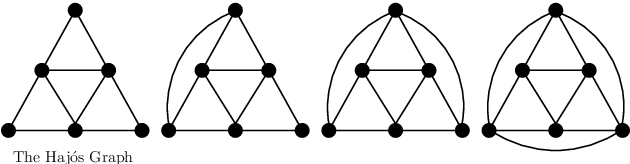•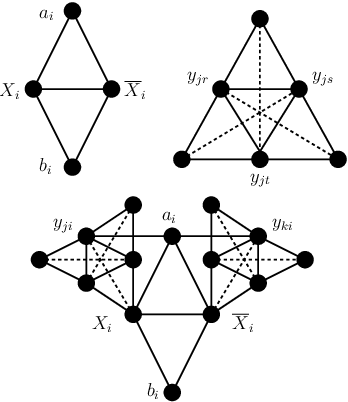•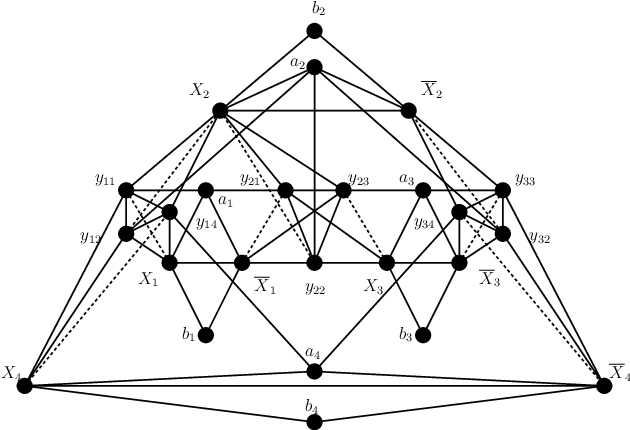•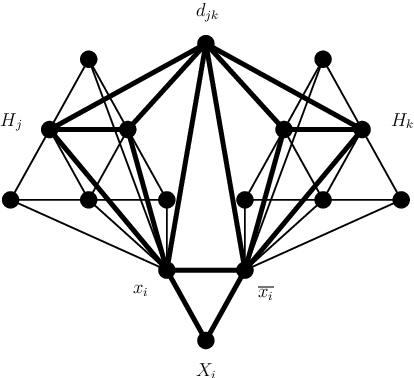•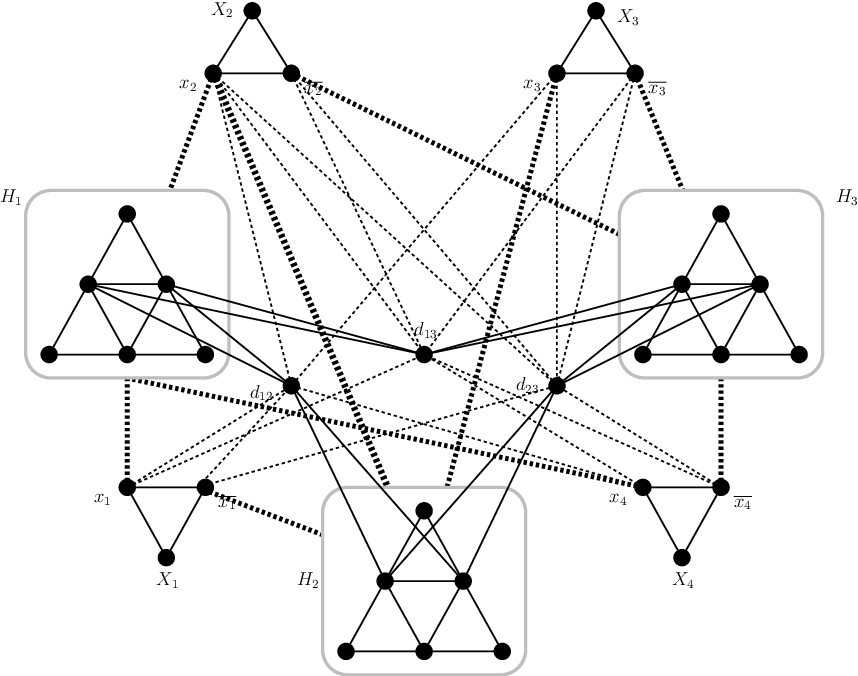Is this relevant?
2009
2009
• Discret. Math.
• 2009
• Corpus ID: 13962364
The P"4-sparse Graph Sandwich Problem asks, given two graphs G^1=(V,E^1) and G^2=(V,E^2), whether there exists a graph G=(V,E… Expand
Is this relevant?
2007
2007
• International Multi-Conference on Computing in…
• 2007
• Corpus ID: 16920753
The stubborn partition is a partition of the vertex set of a graph G into at most four parts A, B, C, D, with the following… Expand
•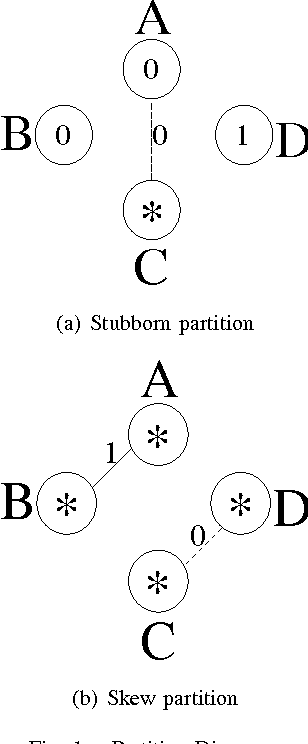•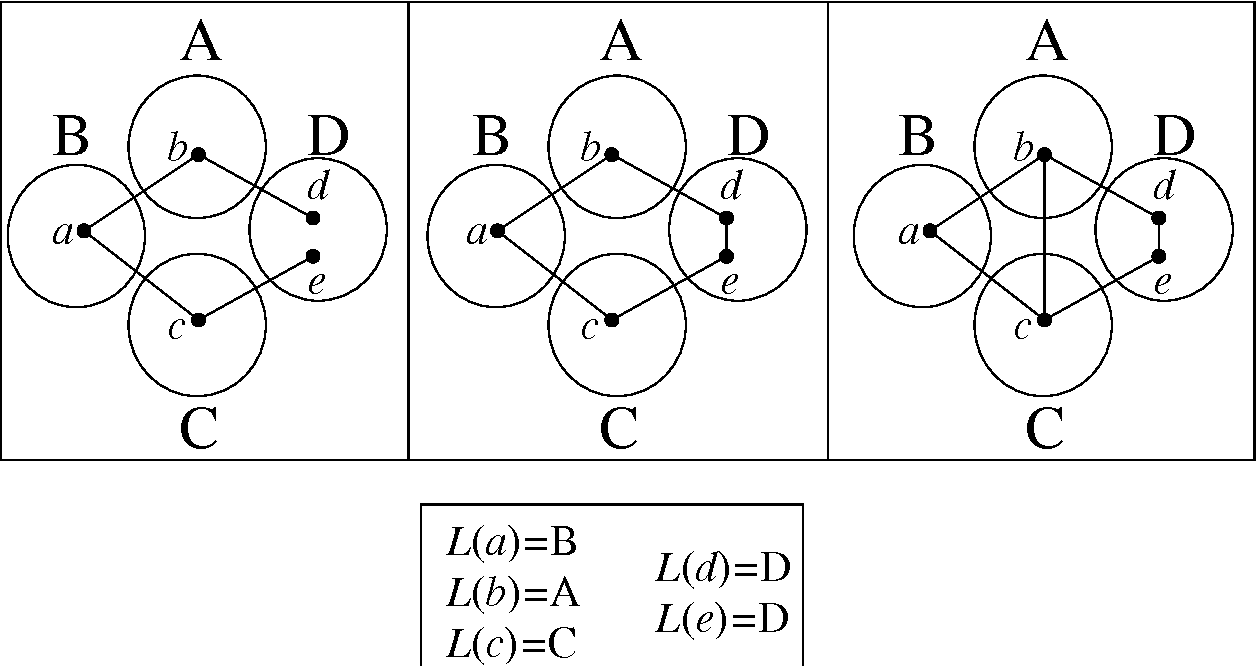•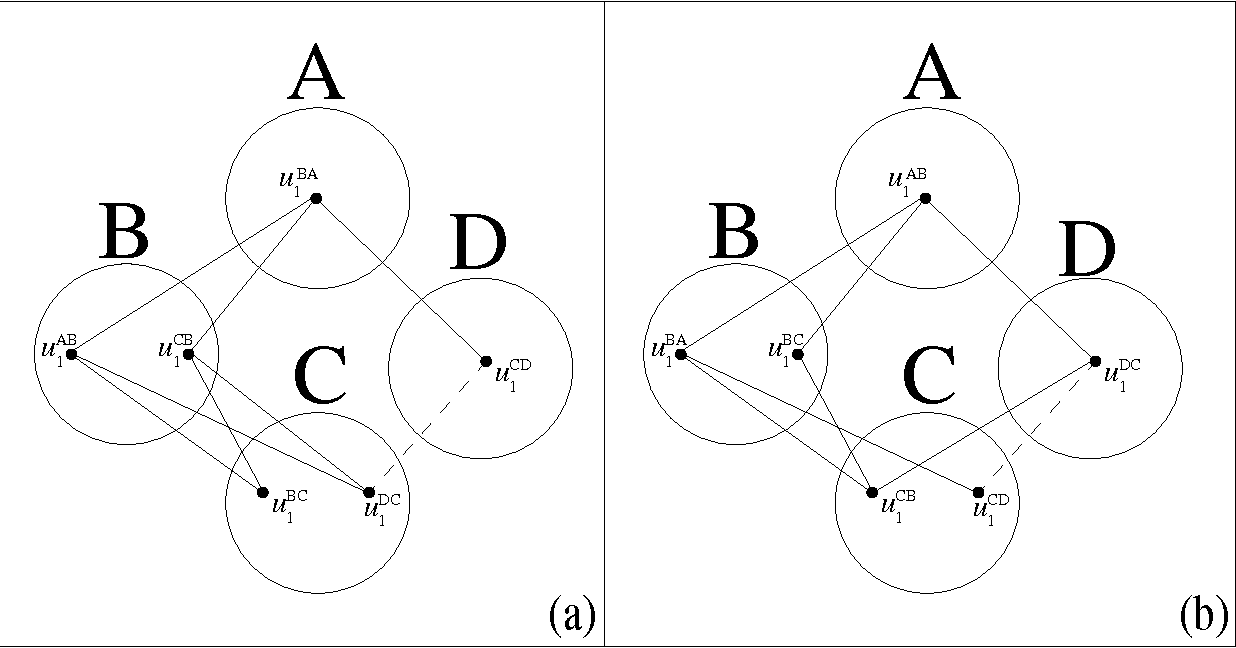•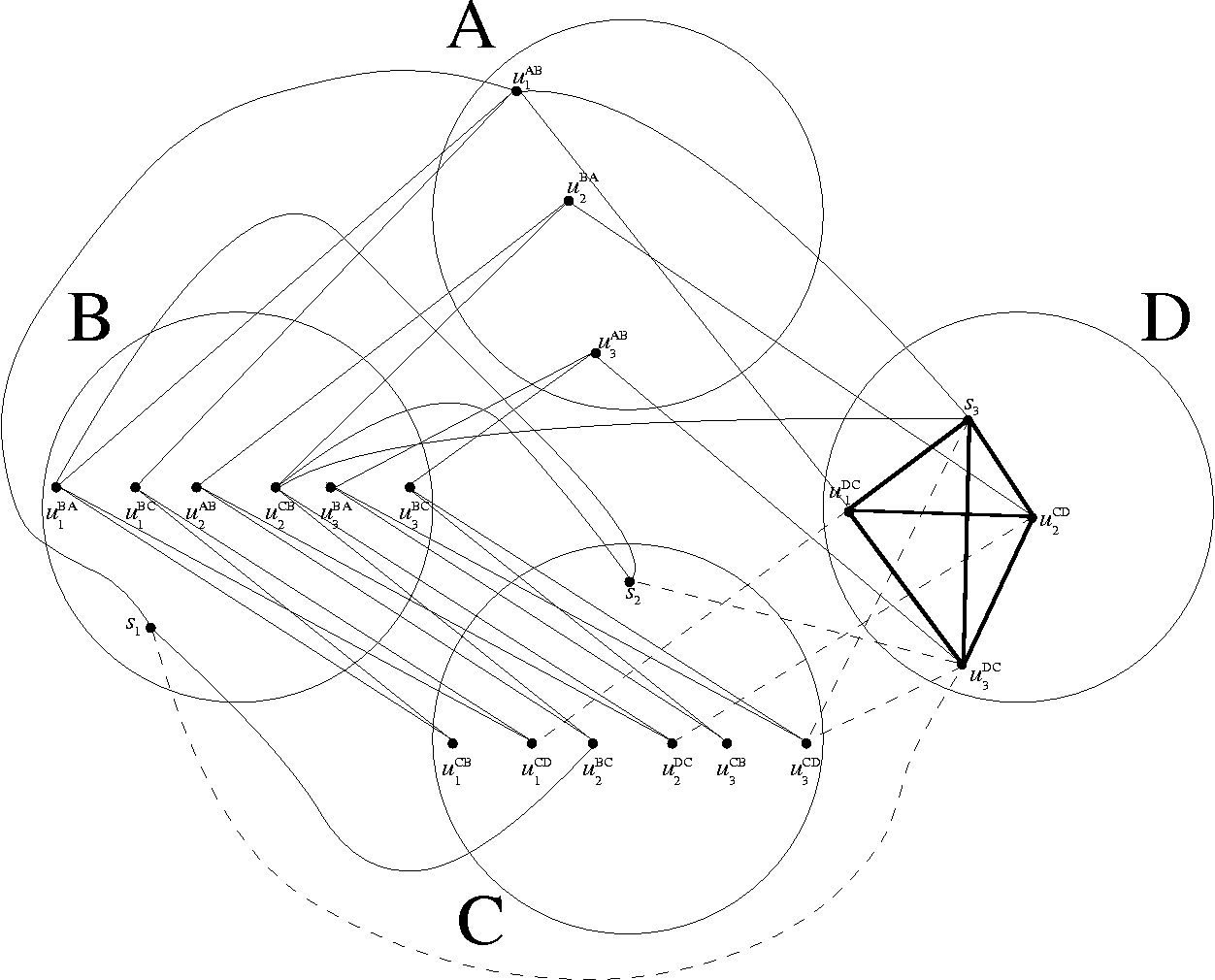Is this relevant?
2005
2005
• Electron. Notes Discret. Math.
• 2005
• Corpus ID: 41284141
Abstract The P 4 -sparse Graph Sandwich Problem asks, given two graphs G 1 = ( V , E 1 ) and G 2 = ( V , E 2 ) , whether there… Expand
Is this relevant?
2004
2004
• Discret. Appl. Math.
• 2004
• Corpus ID: 28425213
A graph G is (k,l) if its vertex set can be partitioned into at most k independent sets and l cliques. The (k,l)-Graph Sandwich… Expand
Is this relevant?
1998
1998
• Graphs Comb.
• 1998
• Corpus ID: 45576341
Abstract. The ℱ Hypergraph Sandwich Problem (ℱHSP) is introduced here as follows: Given two hypergraphs H1=(X,ℰ1) and H2=(X,ℰ2… Expand
Is this relevant?
Highly Cited
1995
Highly Cited
1995
Abstract The graph sandwich problem for property Π is defined as follows: Given two graphs G 1 = ( V , E 1 ) and G 2 = ( V , E 2… Expand
•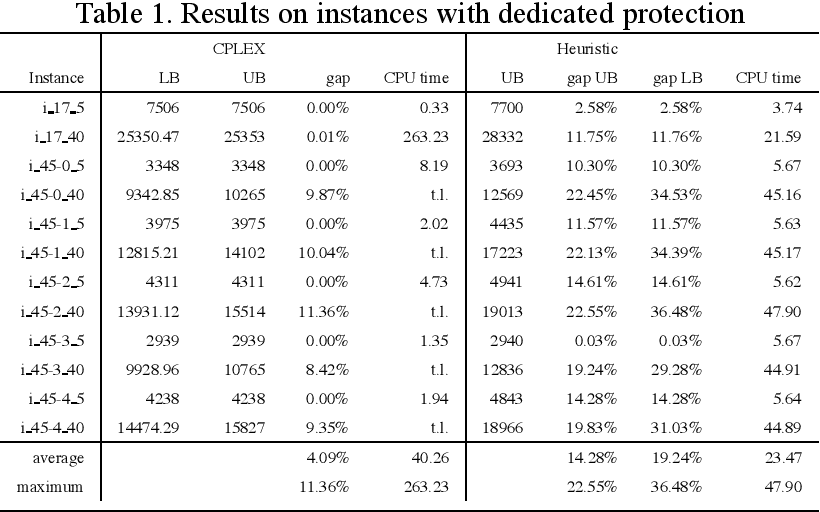•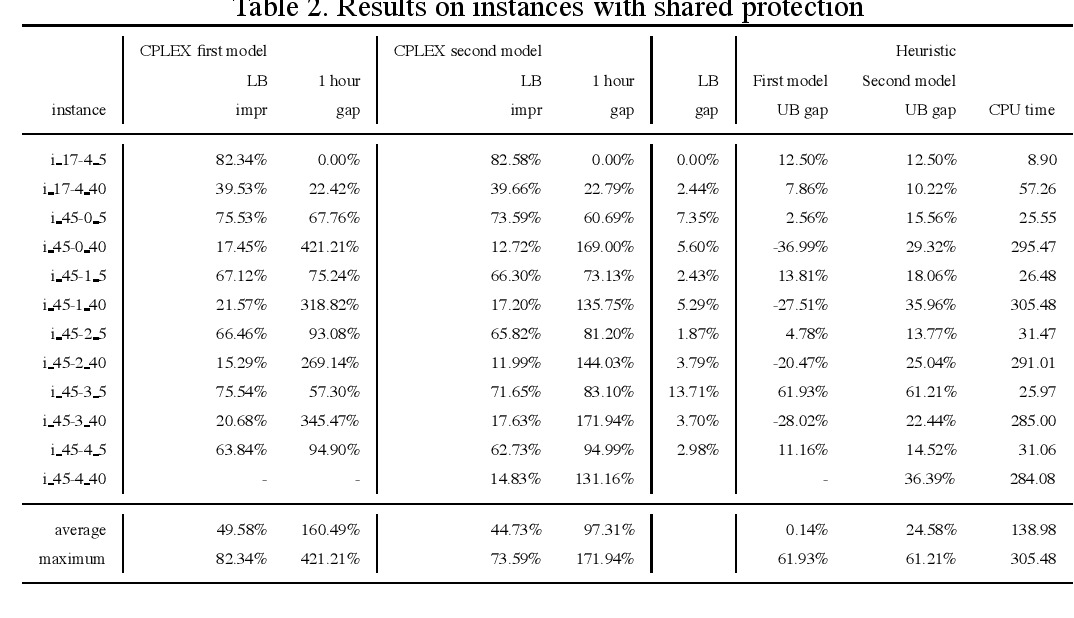•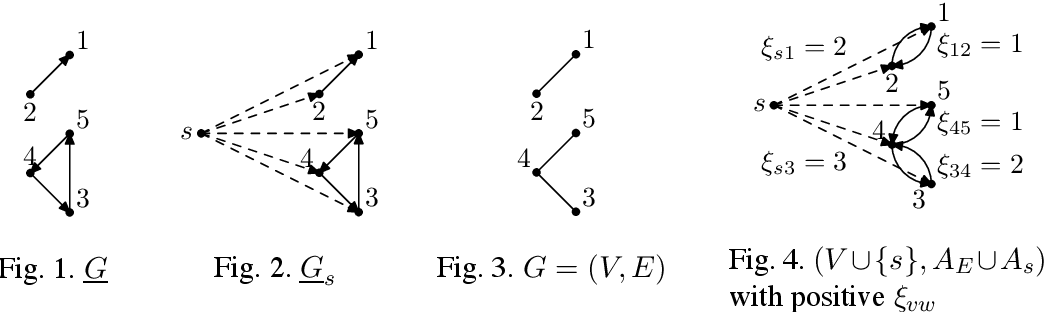•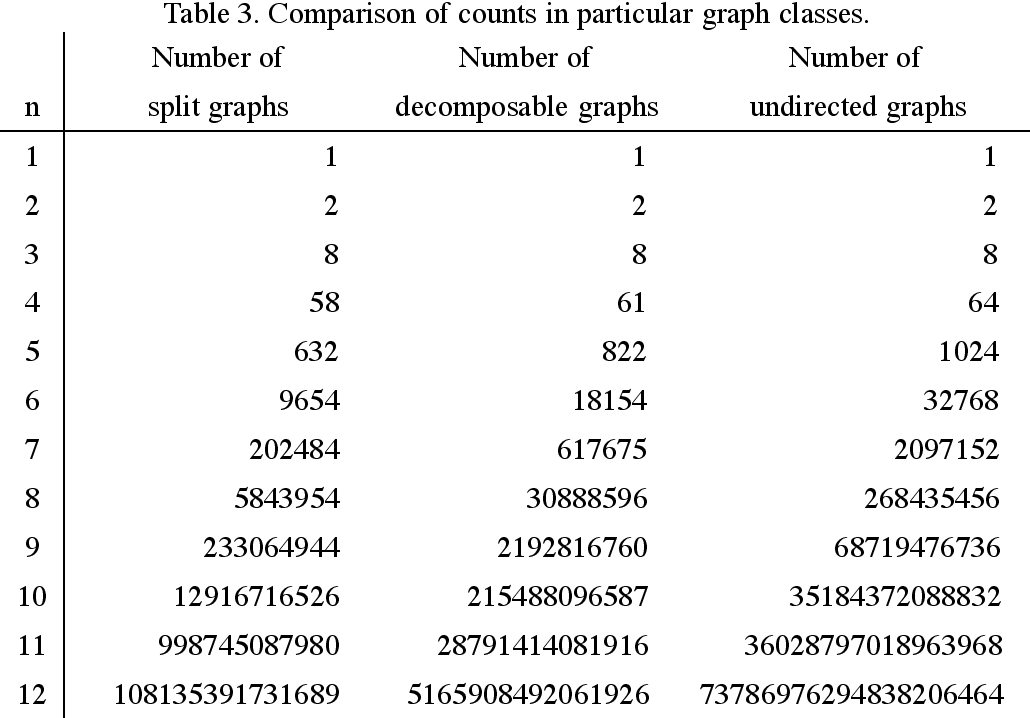•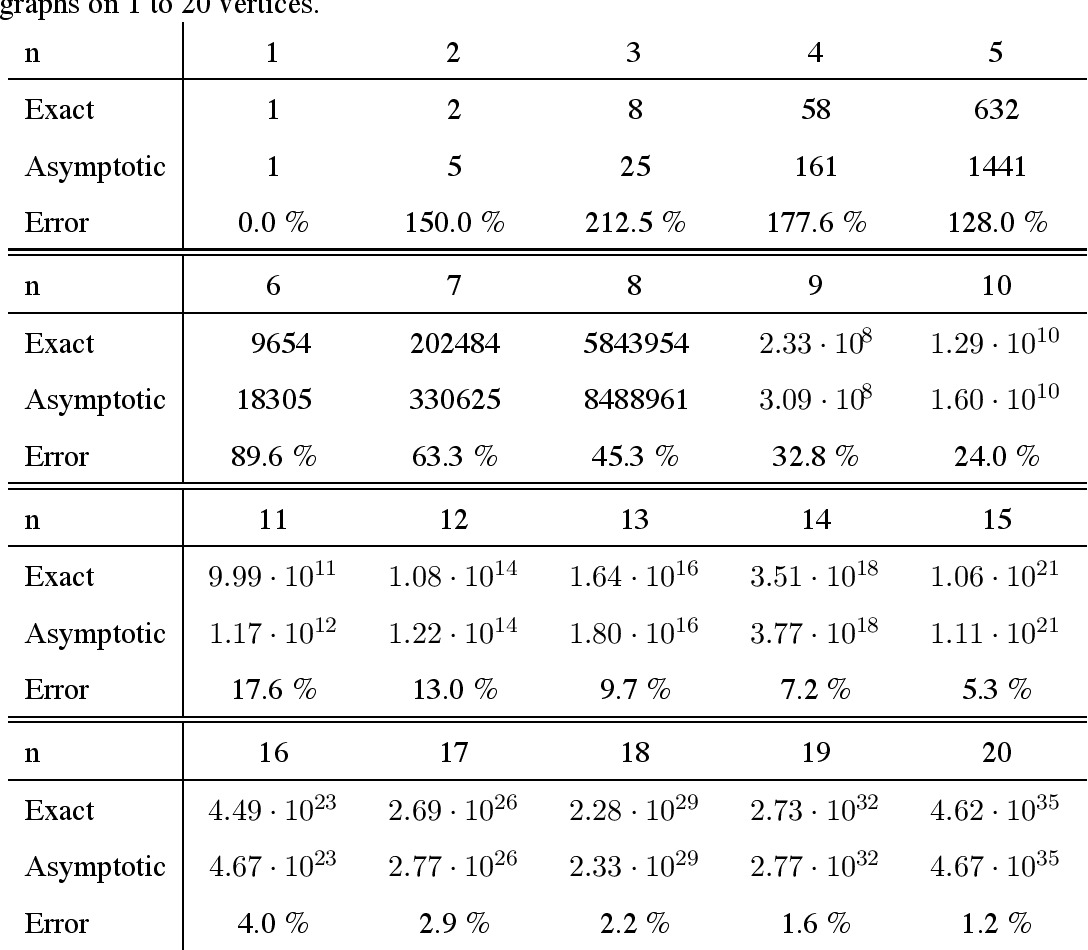Is this relevant?
Highly Cited
1993
Highly Cited
1993
• JACM
• 1993
• Corpus ID: 15708027
Temporal events are regarded here as intervals on a time line. This paper deals with problems in reasoning about such intervals… Expand
Is this relevant?